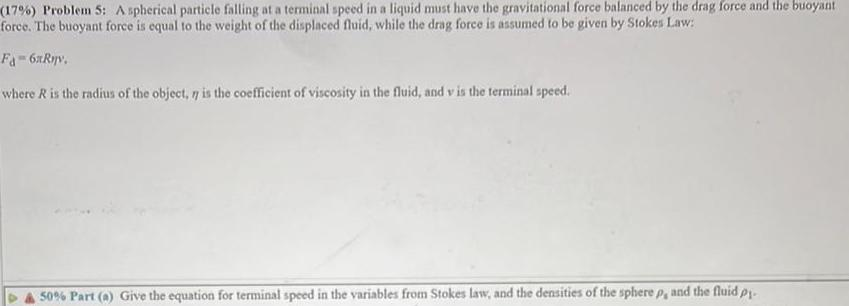Question:

# A spherical particle falling at a terminal speed in aA spherical particle falling at a terminal speed in a liquid must have the gravitational force balanced by the drag force and the buoyant force. The buoyant force is equal to the weight of the displaced fluid, while the drag force is assumed to be given by Stokes Law: Fd=6xRny. where R is the radius of the object, 7 is the coefficient of viscosity in the fluid, and v is the terminal speed. 50% Part (a) Give the equation for terminal speed in the variables from Stokes law, and the densities of the sphere p, and the fluid pj.